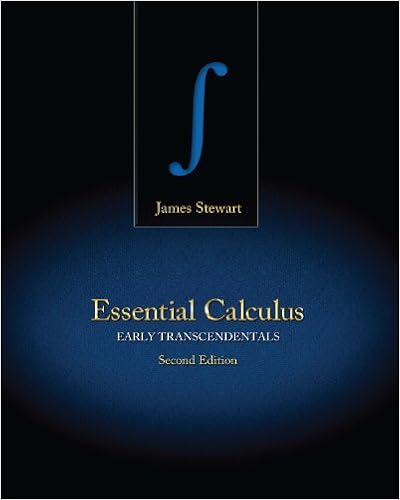# Connected to any drive shaft so we usually dont care

• 56

This preview shows page 20 - 22 out of 56 pages.

##### We have textbook solutions for you!
The document you are viewing contains questions related to this textbook.The document you are viewing contains questions related to this textbook.
Chapter 10 / Exercise 18
Essential Calculus: Early Transcendentals
StewartExpert Verified
connected to any drive shaft, so we usually don’t care very much about its angular speed, but we will need to find zP ω to solve for the unknown one of zS ω , zPC ω , zR ω . This seems a terribly difficult problem, but it can be solved in a very simple way with a trick. We start by solving a simpler version of the problem. Suppose that the planet carrier is stationary ( zPC ω =0) and the sun gear rotates with angular speed zS ω (see the animation) . What is the angular velocity of the ring gear? The sun gear and the planet gear are just a standard gear pair so we know that S zS S zP zP zS R R r r ω ω ω ω = = The two touching points on the planet gear and the ring gear must have the same velocity, so (using the rotating gear formula) zP zR R R zP R r r R R ω ω ω ω = = j j We can eliminate zP ω to get the answer: S zR zS R R R ω ω = − Now let’s try the harder problem. The animation (see the html version) shows a general situation, where zS ω , zPC ω are both nonzero. How can we find zR ω now? This is difficult to analyze because the center of the planet gear is not fixed, so it’s hard for us to visualize the motion, and the standard gear formulas don’t work. But we can simplify the problem by analyzing motion in a reference frame that rotates with the planet carrier. For example, imagine attaching a videocamera to the planet carrier – this camera would show the planet carrier to be stationary, with the surrounding world rotating ω Ring gear r Sun gear R S R R zS ω zR Planet Carrier Planet gear ω zP ω zPC
##### We have textbook solutions for you!
The document you are viewing contains questions related to this textbook.The document you are viewing contains questions related to this textbook.
Chapter 10 / Exercise 18
Essential Calculus: Early Transcendentals
StewartExpert Verified